# The weighted average cost of capital

This entry is part 1 of 2 in the series The Weighted Average Cost of Capital

The weighted cost of capital (WACC) and the return on invested capital (ROIC) are the most important elements in company valuation, and the basis for most strategy and performance evaluation methods.

WACC is the discount rate (time value of money) used to convert expected future cash flow into present value for all investors. Usually it is calculated both assuming a constant cost of capital and a fixed set of target market value weights1 , throughout the time frame of the analysis. As this simplifies the calculations, it also imposes severe restrictions on how a company’s financial strategy can be simulated.

Now, to be able to calculate WACC we need to know the value of the company, but to calculate that value we need to know WACC. So we have a circularity problem involving the simultaneous solution of WACC and company value.

In addition all the variables and parameters determining the company value will be stochastic, either by themselves or by being functions of other stochastic variables. As such WACC is a stochastic variable– determined by the probability distributions for yield curves, exchange rates, sale, prices, costs and investments. But this also enables us – by Monte Carlo simulation –to estimate a confidence interval for WACC.

Some researchers have claimed that the free cash flow value only in special cases will be equal to the economic profit value. By solving the simultaneous equations, giving a different WACC for every period, we will always satisfy the identity between free cash flow and economic profit value. In fact we will use this to check that the calculations are consistent.

We will use the most probable value for variables/parameters in the calculations. Since most of the probability distributions involved are non-symmetric (sale, prices etc), the expected values will in general not be equal to the most probable values. And as we shall see, this is also the case for the individual values of WACC.

### WACC

To be consistent with the free cash flow or economic profit approach, the estimated cost of capital must comprise a weighted average of the marginal cost of all sources of capital that involves cash payment – excluding non-interest bearing liabilities (in simple form):

WACC =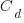= Pre-tax debt nominal interest rate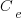= Opportunity cost of equity,
t = Corporate marginal tax rate
D = Market value debt
E = Market value of equity
V = Market value of entity (V=D+E).

The weights used in the calculation are the ratio between the market value of each type of debt and equity in the capital structure, and the market value of the company. To estimate WACC we then first need to establish the opportunity cost of equity and non-equity financing and then the market value weights for the capital structure.

### THE OPPORTUNITY COST OF EQUITY AND NON-EQUITY FINANCING

To have a consistent WACC, the estimated cost of capital must:

1. Use interest rates and cost of equity of new financing at current market rates,
2. Be computed after corporate taxes,
3. Be adjusted for systematic risk born by each provider of capital,
4. Use nominal rates built from real rates and expected inflation.

However we need to forecast the future risk free rates. They can usually be found from the yield curve for treasury notes, by calculating the implicit forward rates.

### THE OPPORTUNITY COST OF EQUITY

The equation for the cost of equity (pre investor tax), using the capital asset pricing model (CAPM) is:

C =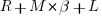R  = risk-free rate,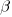= the levered systematic risk of equity,

If tax on dividend and interest income differs, the risk-free rate and the market premium has to be adjusted, assuming tax rate -ti, for interest income:

R = (1-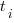)*R  and  M = M+*R.= Investor tax rate,
R  = tax adjusted risk-free rate,

The pre-tax cost of equity can then be computed as: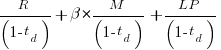(pre-tax) =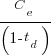=Where the first line applies for an investor with a tax rate of -td, on capital income, the second line for an investor when tax on dividend and interest differs 2 .

The long-term strategy is a debt-equity ratio of one, the un-levered beta is assumed to be 1.1 and the market risk premium 5.5%. The corporate tax rate is 28%, and the company pays all taxes on dividend. The company’s stock has low liquidity, and a liquidity premium of 2% has been added.In the Monte Carlo simulation all data in the tables will be recalculated for every trial (simulation), and in the end produce the basis for estimating the probability distributions for the variables. This approach will in fact create a probability distribution for every variable in the profit and loss account as well as in the balance sheet.

### THE OPPORTUNITY COST OF DEBT

It is assumed that the pre-tax debt interest rate can be calculated using risk adjusted return on capital (RAROC) as follows:

Lenders Cost =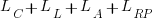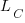= Lenders Funding Cost (0.5%),= Lenders Average Expected Loss (1.5%),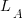= Lenders Administration Cost (0.8%),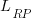= Lenders Risk Premium (0.5%).

The parameters (and volatility) have to be estimated for the different types of debt involved. In this case there are two types; short -term with a maturity of four years and long-term with a maturity of 10 years. The risk free rates are taken from the implicit forward rates in the yield curve and lenders cost are set to 3.3%.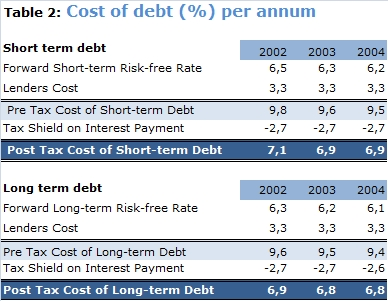In every period the cost and value of debt are recalculated using the current rates for that maturity, ensuring use of the current (future) opportunity cost of debt.

### THE MARKET VALUE WEIGHTS

By solving the simultaneous equations, we find the market value for each type of debt and equity:And the value weights:Multiplying the value weights by the respective rate and adding, give us the periodic most probable WACC rate: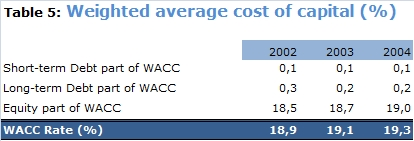As can be seen from the table above, the rate varies slightly from year to year. The relative small differences are mainly due to the low gearing in the forecast period.

### MONTE CARLO SIMULATION

In the figure below we have shown the result from simulation of the company’s operations, and the resulting WACC for year 2002. This shows that the expected value of WACC in is 17.4 %, compared with the most probable value of 18.9 %. This indicates that the company will need more capital in the future, and that an increasing part will be financed by debt. A graph of the probability distributions for the yearly capital transactions (debt and equity) in the forecast period would have confirmed this.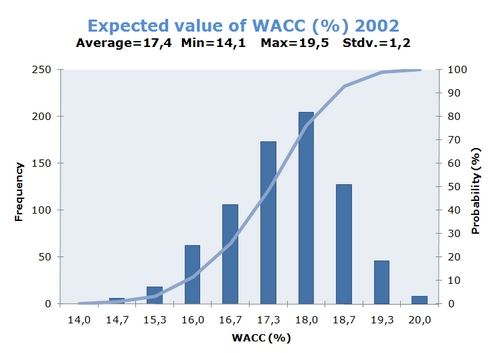In the figure the red curve indicates the cumulative probability distribution for the value of WACC in this period and the blue columns the frequencies. By drawing horizontal lines on the probability axis (left), we can find confidence intervals for WACC. In this case there is only a 5% probability that WACC will be less than 15%, and a 95% probability that it will be less than 20%. So we can expect WACC for 2002 with 90% probability to fall between 15% and 20%. The variation is quite high  – with a coefficient of variation of 6.83.

### VALUATION

The value of the company and the resulting value of equity can be calculated using either the free cash flow or the economic profit approach. Correctly done, both give the same value. This is the final test for consistency in the business model. The calculations are given in the tables below, and calculated as the value at end of every year in the forecast period.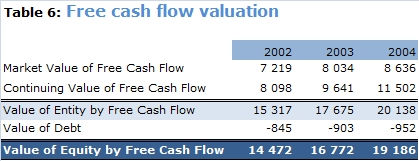As usual, the market value of free cash flow is the discounted value of the yearly free cash flow in the forecast period, while the continuing value is the value of continued operation after the forecast period. All surplus cash are paid, as dividend so there is no excess marketable securities.The company started operations in 2002 after having made the initial investments. The charge on capital is the WACC rate multiplied by the value of invested capital. In this case capital at beginning of each period is used, but average capital or capital at end could have been used with a suitable definition of capital charge.
Economic profit has been calculated by multiplying RIOC – WACC with invested capital, and the market value at any period is the net present value of future economic profit. The value of debt as the net present value of future debt payments – is equal for both methods.

For both methods using the same series of WACC when discounting cash the flows, we find the same value for the both company and equity. This ensures that the calculations are both correct and consistent.

Tore Olafsen and John Martin Dervå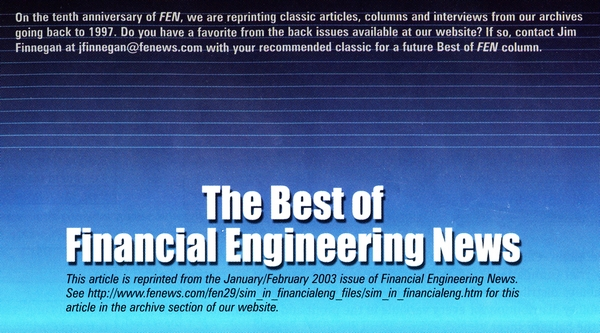Series NavigationWACC, Uncertainty and Infrastructure Regulation >>
1. Valuation, Measuring and Managing the Value of Companies. Tom Copeland et al. []
2. See also: Wacc and a Generalized Tax Code, Sven Husmann et al.,  Diskussionspapier 243 (2001), Universität Hannover []
3. Coefficient of variation = 100*st.dev/mean []S@R develops models for support of decision making under uncertainty. Taking advantage of recognized financial and economic theory, we customize simulation models to fit specific industries, situations and needs.

### 2 Enlightened Replies

1.PALIARD says:

Hello

I am somehow puzzled : how can the WACC be larger than the cost of equity, since the cost of debt is lower ?

2.Tore Olafsen says:

Thank you for your comment and for pointing out the error. The table gave the un-levered cost of equity instead of the levered cost of equity. The error is corrected and the full and detailed calculation is now attached as a PDF file.

Top